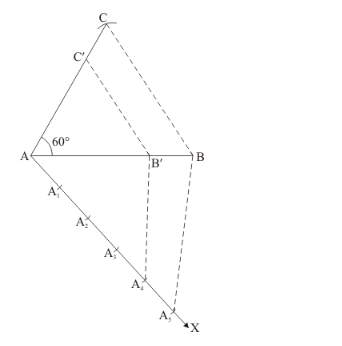# Construct a triangle similar to Δ ABC in which AB = 4.6 cm,

Question:

Construct a triangle similar to Δ ABC in which AB = 4.6 cm, BC = 5.1 cm, ∠A = 60° with scale factor 4 : 5.

Solution:

Given that

Construct a $\triangle A B C$ of given data, $A B=4.6 \mathrm{~cm}, B C=5.1 \mathrm{~cm}$ and $\angle A=60^{\circ}$ and then a triangle similar to it whose sides are $(4: 5=4 / 5)^{\text {th }}$ of the corresponding sides of $\triangle A B C$.

We follow the following steps to construct the givenStep of construction

Step: I- First of all we draw a line segment $A B=4.6 \mathrm{~cm}$.

Step: II- With $A$ as centre draw an angle $\angle A=60^{\circ}$.

Step: III- With $B$ as centre and radius $=B C=5.1 \mathrm{~cm}$, draw an arc, intersecting the arc drawn in step II at $C$.

Step: IV- Joins $B C$ to obtain $\triangle A B C$.

Step: V-Below $A B$, makes an acute angle $\angle B A X=60^{\circ}$.

Step: VI- Along $A X$, mark off five points $A_{1}, A_{2} A_{3} A_{4}$ and $A_{5}$ such that $A A_{1}=A_{1} A_{2}=A_{2} A_{3}=A_{3} A_{4}=A_{4} A_{5}$

Step: VII- Join $A_{5} B$.

Step: VIII- Since we have to construct a triangle each of whose sides is $(4 / 5)^{\text {th }}$ of the corresponding sides of $\triangle A B C$.

So, we take four parts out of five equal parts on $A X$ from point $A_{4}$ draw $A_{4} B^{\prime} \| A_{5} B$, and meeting $A B$ at $B$ '.

Step: IX- From $B^{\prime}$ draw $B^{\prime} C^{\prime} \| B C$, and meeting $A C$ at $C^{\prime}$

Thus, $\triangle A B^{\prime} C^{\prime}$ is the required triangle, each of whose sides is $(4 / 5)^{\text {th }}$ of the corresponding sides of $\triangle A B C$.